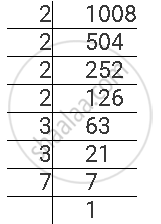# For the Following Number, Find the Smallest Whole Number by Which It Should Be Multiplied So as to Get a Perfect Square Number. Also Find the Square Root of the Square Number So Obtained. 1008 - Mathematics

For the following number, find the smallest whole number by which it should be multiplied so as to get a perfect square number. Also find the square root of the square number so obtained.

1008

#### Solution

1008 can be factorised as follows.1008 = 2 × 2 × 2 × 2 × 3 × 3 × 7

Here, prime factor 7 does not have its pair. If 7 gets a pair, then the number will become a perfect square. Therefore, 1008 can be multiplied with 7 to obtain a perfect square.

1008 × 7 = 7056 = 2 × 2 ×2 × 2 × 3 × 3 × 7 × 7

Therefore, 1008 × 7 = 7056 is a perfect square.

∴ sqrt(7056) = 2 x 2 x 3 x 7 = 84

Concept: Finding Square Root Through Prime Factorisation
Is there an error in this question or solution?

#### APPEARS IN

NCERT Class 8 Maths
Chapter 6 Squares and Square Roots
Exercise 6.3 | Q 5.3 | Page 102